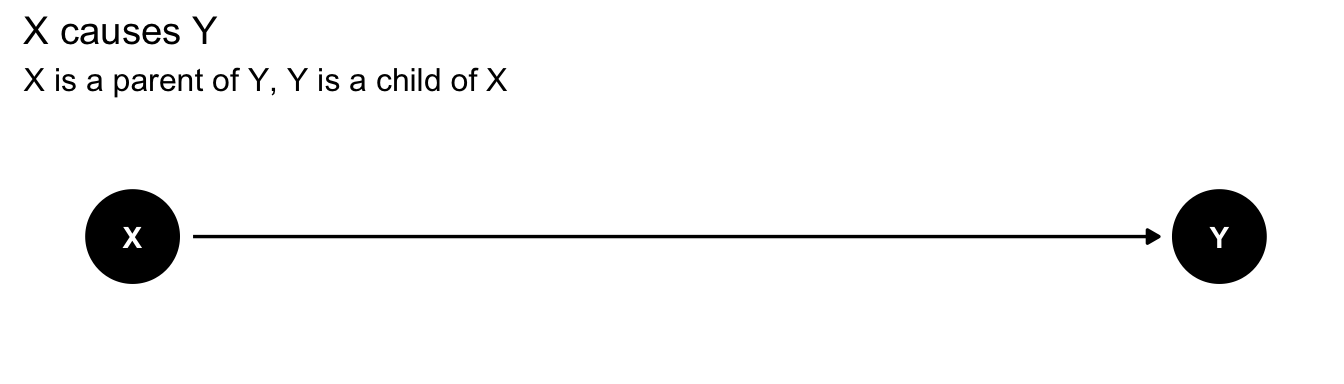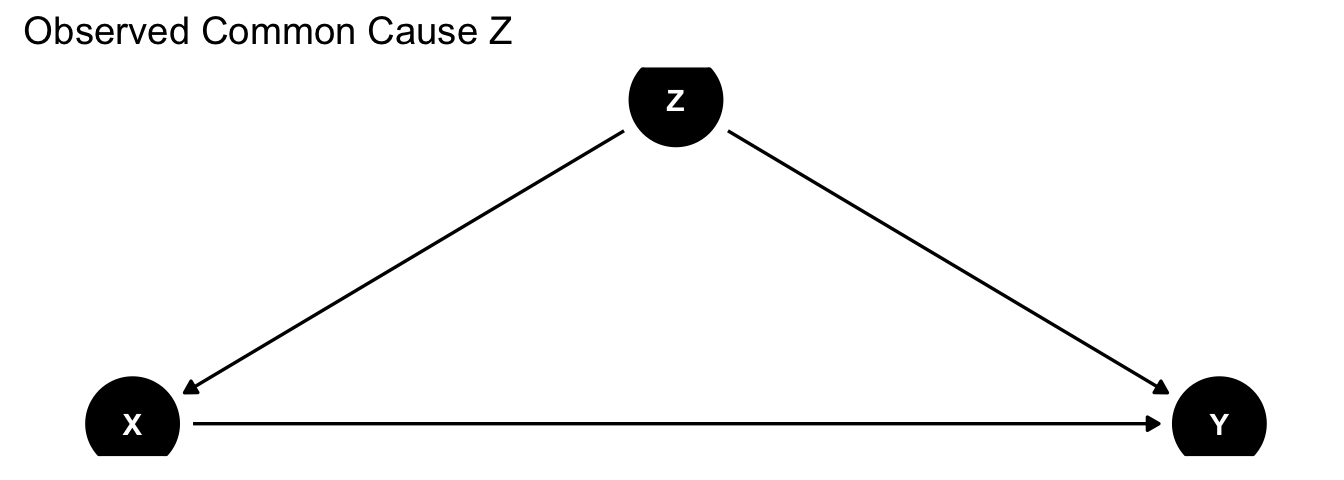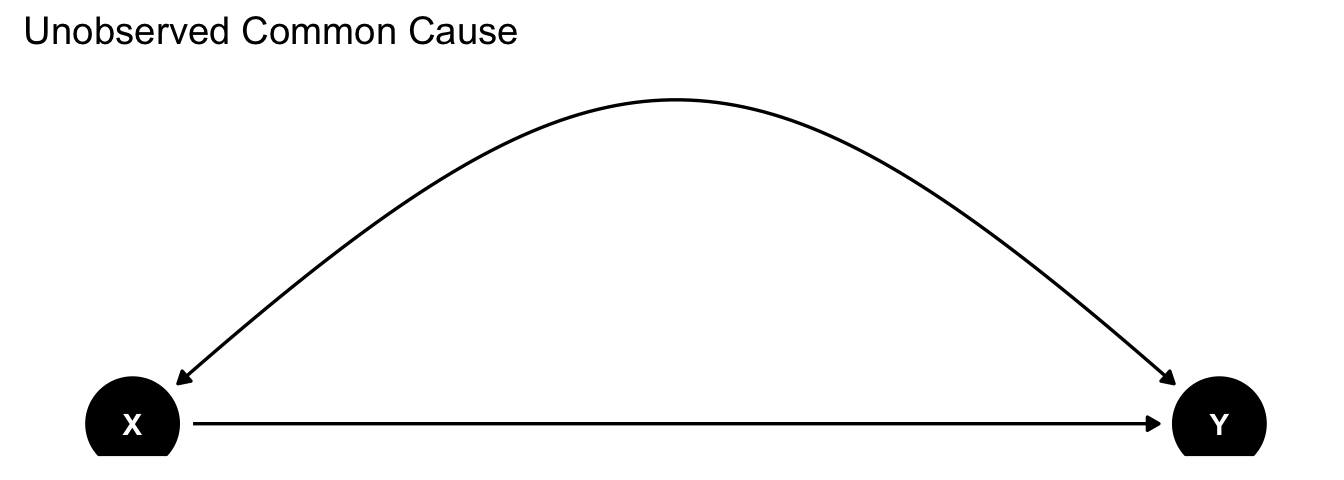## Causality: What is it?

• Possible worlds
• Invariance
• Principles of comparative statics

## Causal Hierarchy

• “Correlation is not causation”
• Almost all observational distributions are compatible with multiple interventional distributions
• “Interventions are not counterfactuals”
• Almost all interventional distributions are compatible with multiple counterfactual distributions
• Implication: cannot extrapolate from lower to higher level of causal hierarchy without causal assumptions
• No inference on effects of policies using observational data without claims about what would happen in an experiment
• Running an experiment is one good way of justifiably making such claims!
• No inference on counterfactual state of individuals from observational OR experimental data without assumptions on individual counterfactual states
• “Fundamental problem of causal inference”: Don’t ever see the counterfactual
• Welfare analysis fundamentally requires untestable economic assumptions
• Maybe these are fine! We know from physical or economic principle what somebody would have done
• Or maybe not: why depend on theory you can’t verify?

## Structural Causal Model (SCM)

• A canonical structural model of causal interactions between variables
• Imposes only qualitative restriction of which variables cause which other variables
• Each endogenous variable $$(Y_1,\ldots,Y_J)$$ is described by a structural equation $Y_1=f_1(Y_2,\ldots,Y_p,U_1)$ $Y_2=f_2(Y_1,Y_3,\ldots,Y_p,U_2)$ $\vdots$ $Y_p=f_p(Y_1,Y_2,\ldots,Y_{p-1},U_p)$
• Each structural equation contains only direct causes
• Variables $$Y_j$$ with no effect are excluded
• Variables $$U$$ are exogenous
• Not caused by any variable $$Y$$ in system
• Represent any source of possibly idiosyncratic variation or heterogeneity in each realization of a particular variable
• We will, unless otherwise specified, assume Independent Errors assumption
• $$(U_1,\ldots,U_p)$$ are drawn from mutually independent joint distribution $$\Pi_{j=1}^{p}F_{U_j}$$

## Causal Implications of SCM

• Observational distribution $$P(Y_1,\ldots,Y_p)$$ is joint distribution of $$(Y_1,\ldots,Y_p)$$ consistent with system of equations and joint distribution of $$U_j$$
• Call map from $$(U_1,\ldots,U_p)$$ to $$(Y_1,\ldots,Y_p)$$ the reduced form of the system, and the pushforward of $$\Pi_{j=1}^{p}F_{U_j}$$ through this map the observational distribution
• Reduced form exists and is unique under set of restrictions described below
• Intervention described by process of surgery:
• Replace $$f_j$$ by a fixed value $$y$$, then define new reduced from distribution consistent with modified equations and same distribution of $$U$$
• Distribution of $$(Y_1,\ldots,Y_{j-1},y,Y_{j+1},\ldots,Y_p)$$ is called $$P(Y_{-j}|do(Y_j=y))$$
• To keep track of variables under intervention, denote them as “potential outcomes”
• What would this variable be if we were to set $$Y_j$$ to $$y$$
• Will see this denoted as $$Y_i(Y_j=y)$$, $$Y_i^{Y_j=y}$$
• If it is clear from context which variable is being intervened on, will also see $$Y_i(y)$$ or $$Y_i^{y}$$
• “First law of causal inference”: $$Y_i^{Y_j=y}$$ is value of $$Y_i$$ in model where equation for $$Y_j$$ replaced
• All technical results are just shortcuts for performing this calculation
• If we assume that observational and interventional distributions are functions of same realization of $$U$$, can define a joint distribution as pushforward through Cartesian product of reduced forms
• Call any function of this joint distribution a counterfactual
• E.g. $$P(Y_i(Y_j=y)|Y_i=z)$$ "What is distribution of $$Y_i$$ in the world where we set $$Y_j$$ to $$y$$ among units for which $$Y_i$$ otherwise would have been $$z$$?

## Causal graphs

• Because nonparametric model above imposes no restrictions on form of relationship between variables aside from fact of which are connected, can express model as a graph
• Variables are nodes or vertices: box or circle
• $$V$$ is a set $$\{1,\ldots,p\}$$
• Direct causal effects are (directed) edges
• $$E=\{(i,j)\in V\times V: Y_i\text{ appears in }f_j \}$$
• Lines with arrows indicating direction
• Nodes at source are called parents: $$Par(V_i)=\{j\in V: (j,i)\in E\}$$
• Nodes at ends are children $$Chil(V_i)=\{j\in V: (i,j)\in E\}$$
• Sequence of connected arrows is a path - It is directed if arrows all point in direction of sequence
• Simplest example: structural equation model
• $$Y=f_1(X,U_1)$$ $$X=f_2(U_2)$$
• Graph by R packages dagitty and ggdag
library(dagitty) #Library to create and analyze causal graphs
suppressWarnings(suppressMessages(library(ggdag))) #library to plot causal graphs
yxdag<-dagify(Y~X) #create graph with arrow from X to Y
#Set position of nodes so they lie on a straight line
coords<-list(x=c(X = 0, Y = 1),y=c(X = 0, Y = 0))
coords_df<-coords2df(coords)
coordinates(yxdag)<-coords2list(coords_df)
ggdag(yxdag)+theme_dag_blank()+labs(title="X causes Y",subtitle="X is a parent of Y, Y is a child of X") #Plot causal graph• $$U_1,U_2$$ not shown explicitly: $$X$$ and $$Y$$ are variables of interest, with exogenous random variation caused by $$U_1,U_2$$

## Direced Acyclic Graphs

• Common to add assumption that structural equations are acyclic
• Causal graph has no directed path from a variable to itself
• Variables do not cause themselves
• Ensures SCM delivers distributions which exist and are unique
• Reduced form can be produced constructively by recursive substitution
• Substitute variable in direction of arrows for its structural equation
• Repeat until no endogenous variable appears on righthand side
• For acyclic SCM with independent errors, we have fully complete understanding of identification of any feature of interventional or counterfactual distributions
• Defined using “do-calculus” and automated in software: more in later classes
• Refer to models of this form as “Directed Acyclic Graphs” or DAGs

## Independent Errors

• Independence allows interpretation of $$U_j$$ as variation just in $$Y_j$$
• Restrictive only if we require all endogenous variables to be observed
• If some $$\mathcal{J}\subset\{1\ldots p\}$$ variables are unobserved, common variation between observed variables can be modeled by presence of linked unobserved variable(s)
• Reflects principle that all correlation derives from some causal factors
• Graph then represents distribution of observed and unobserved variables
• Common to work with subgraph containing only observed variables
• Unobserved common causes represented by bidirected arrows
• Can work with such correlated errors models directly, but since properties derivable from marginalizing DAG, will primarily work just with independent case
yxzdag<-dagify(Y~X+Z, X~Z) #create graph with arrow from X to Y
#Set position of nodes so they lie on a straight line
coords<-list(x=c(X = 0, Y = 1, Z=0.5),y=c(X = 0, Y = 0, Z=0.5))
coords_df<-coords2df(coords)
coordinates(yxzdag)<-coords2list(coords_df)
ggdag(yxzdag)+theme_dag_blank()+labs(title="Observed Common Cause Z") #Plot causal graphyxydag<-dagitty("dag{Y<->X; X->Y}") #create graph with arrow from X to Y
#Set position of nodes so they lie on a straight line
coords<-list(x=c(X = 0, Y = 1),y=c(X = 0, Y = 0))
coords_df<-coords2df(coords)
coordinates(yxydag)<-coords2list(coords_df)
ggdag(yxydag)+theme_dag_blank()+labs(title="Unobserved Common Cause") #Plot causal graph## Limitations of DAG framework

• Acyclicity rules out models defined by simultaneous restrictions: e.g. equilibrium
• Directed structure requires variables to have explicit causal characterization in terms of other variables
• Rules out models defined by implicit characterizations: e.g. optimality
• But who needs those? Not, apparently, applied microeconomists.
• Identification results may however be insufficient because we have more information
• Monotonicity, linearity, (non)-Gaussianity etc may provide additional identifying information
• Ongoing research on algorithmic characterization of identified features in models with additional assumptions
• Results may also be inapplicable if we have less information than needed to uniquely define model
• Usually only confident about small subset of relationships
• Ongoing research on characterization of identified features under collection of all plausible models

## Potential Outcomes

• Common in applied econometrics to avoid explicit reference to structural functions altogether
• Instead, take assumptions on potential outcomes as primitives
• For a particular intervention setting $$X$$ to $$x$$, observations $$\{Y,\{Y^{x}\}_{x\in\mathcal{X}},X\}$$ are assumed to have a joint distribution
• Realized outcomes $$\{Y,X\}$$ are related to potential outcomes $$\{Y^{X=x}\}_{x\in\mathcal{X}}$$ by the causal consistency assumption
• $$Y=\int Y^{x}\delta_{X=x}dx$$, read "$$Y=Y^x$$ when $$X=x$$
• E.g., binary $$X$$ case $$Y= Y^{1}1\{X=1\}+Y^{0}1\{X=0\}$$
• Additional properties of the distribution may be assumed based on context
• A fully specified structural equation model will imply a joint distribution over all sets of potential outcomes which satisfies consistency
• Converse is also true: if random variables defined on common probability space $$\Omega$$, define structural functions as $$f(x,\omega):=Y^x(\omega)$$
• However, typically only a subset of features of joint distribution will be specified
• Often, only the marginal distributions of counterfactuals are described
• Good reason for this: “fundamental problem of causal inference”
• Never, under any circumstances, observe $$(Y^{X=x_1},Y^{X=x_2})$$ for $$x_1\neq x_2$$
• Joint distribution is not uniquely pinned down without untestable assumptions
• Typically many structural models with exactly same empirical implications in all observational or interventional settings
• Arguably, prudent to start by considering what implications can be drawn only using properties shared by all models which are feasibly distinguishable

## Potential Outcomes and Finite Samples

• Commonly see potential outcomes $$\{Y_i,\{Y_i^{x}\}_{x\in\mathcal{X}},X_i\}_{i=1}^{n}$$ defined only for finite population
• Model is same as above but joint distribution is “empirical distribution” only over $$i=1\ldots n$$
• Uncertainty arises in this model only because not all potential outcomes observed, not from sampling variability
• Sometimes called “design based inference”
• Setting represents cases where extrapolation to larger population is not desired or sensible
• “Suppose we have data on school districts of Minnesota. How does Minnesota go to infinity? By invasion of surrounding states and provinces of Canada, not to mention Lake Superior, and eventually by rocket ships to outer space?” (Geyer (2013))
• “Identification” not usually feasible here, but can perform inference
• Extrapolation is only to same set of districts at same time, but with different policy
• Not actually clear what you can do with that extrapolation: if you change the policy next year, will potential outcomes be exactly the same, or will they be drawn from some distribution (maybe identical, maybe not)?
• Potential outcomes often paired with this framework, but question of limit sequence is distinct from causal modeling question
• Just be aware of distribution over which inference is performed, as standard error formulas change a lot

## Why causality is hard: nonidentifiability

• Common, narrow goal in binary case: Average Treatment Effect of $$X$$ on $$Y$$, $$ATE=E[Y^1-Y^0]$$
• 2 observationally identical data sets, 2 different true values
• Difference in means estimate: $$\widehat{E}[Y_i|X_i=1]-\widehat{E}[Y_i|X_i=0]$$ is 1 for each
$$Y_i^1$$ $$Y_i^0$$ $$X_i$$ $$Y_i$$
1 0 1 1
1 0 1 1
1 0 0 0
1 0 0 0
• ATE = $$\frac{1}{4}\sum_i Y^1_i-Y^0_i=1$$: positive, identical effect
$$Y_i^1$$ $$Y_i^0$$ $$X_i$$ $$Y_i$$
1 1 1 1
1 1 1 1
0 0 0 0
0 0 0 0
• ATE = $$\frac{1}{4}\sum_i Y^1_i-Y^0_i=0$$: no effect, but level associated with treatment
• No estimator can distinguish 2 cases: need additional assumptions
• “Correlation is not causation”

## Nonidentifiability of causal efects

• What went wrong? Systematic relationship of treatment and potential outcomes
• $$E[Y|X=1]-E[Y|X=0]=E[Y^1X+Y^0(1-X)|X=1]-E[Y^1X+Y^0(1-X)|X=0]$$ (consistency)
• $$=E[Y^1|X=1]-E[Y^0|X=0]$$
• $$=(E[Y^1|X=1]-E[Y^0|X=1])+(E[Y^0|X=1]-E[Y^0|X=0])$$
• “Average Treatment on the Treated” + “Selection into treatment”
• Case 1: 1 + 0. Case 2: 0 + 1.
• Because non-observed potential outcome is always missing, may have arbitrary relationship with other variables
• $$Y^x\neg\perp X$$
• Manifestation in structural equations: $$Y^x=f_y(x,Z,U_y)$$ $$X=f_x(Y,Z,U_x)$$
• Happens when $$U_y$$ affects $$X$$ (reverse causation) or $$Z$$ affects both (common cause)

## Experiments

• How to solve: make some assumptions on relationship of observables and unobservables
• Fundamental approach: set $$X$$ yourself
• Ensures you know $$f_x()$$ exactly
• Physical meaning of graph surgery
• Randomization: assign $$X$$ independently of all other characteristics
• $$Y^x\perp X$$ “Random assignment”
• $$E[Y|X=x]=E[Y^1X+Y^0(1-X)|X=x]$$ (consistency)
• $$=E[Y^x|X=1]$$
• $$=E[Y^x]$$ (independence)
• Average treatment effect equals difference in means
• No way to know from data that you are in an experiment: need a priori knowledge

## What experiments don’t identify

• Consider data from experiment: drug $$X$$ given to patient $$i$$ who either lives $$Y=1$$ or dies $$Y=0$$
• ATE identified by difference in means since potential outcome distribution same in treated, untreated groups
$$Y_i^1$$ $$Y_i^0$$ $$X_i$$ $$Y_i$$
1 0 1 1
0 0 1 0
1 1 1 1
1 0 0 0
0 0 0 0
1 1 0 1
• ATE = $$\frac{1}{6}\sum_i Y^1_i-Y^0_i=\frac{1}{N_1}\sum_i Y_i1\{X_i=1\}-\frac{1}{N_0}\sum_iY_i1\{X_i=0\}=\frac{1}{3}$$
• Drug $$X$$ saves life of 1 in 3, no effect on other 2
$$Y_i^1$$ $$Y_i^0$$ $$X_i$$ $$Y_i$$
1 0 1 1
0 1 1 0
1 0 1 1
1 0 0 0
0 1 0 1
1 0 0 0
• ATE = $$\frac{1}{6}\sum_i Y^1_i-Y^0_i=\frac{1}{N_1}\sum_i Y_i1\{X_i=1\}-\frac{1}{N_0}\sum_iY_i1\{X_i=0\}=\frac{1}{3}$$
• Drug $$X$$ saves life of 2 in 3, kills 1
• Any risk-sensitive or minimax welfare measure (eg Hippocratic oath: “first do no harm”) not to mention the patients who are killed, cares deeply about difference between two scenarios.
• But they are observationally indistinguishable, even with an experiment!
• “Interventions are not counterfactuals”

## What’s going on

• Causal hierarchy theorem (Bareinboim et al. (2020))
• Almost all observational distributions can arise from models with distinct interventional effects
• Almost all interventional/experimental distributions can arise from models with distinct counterfactual effects
• Model observational distributions with probability theory
• Draw conclusions about effect of interventions only with causal assumptions such as direct (experimental) assignment of certain variables
• Model interventions with reference to distributions identifiable with experiments
• Draw conclusions about counterfactuals only with knowledge of structural invariances
• Tools exist which sharply distinguish conclusions at 2nd and 3rd levels
• E.g. Hernán and Robins (2020) book for SWIGs, Pearl, Dawid for Causal Bayesian networks
• Practically: work with “program evaluation” tools which (usually) rely on limited set of interventional assumptions only
• “quasiexperimental approach”
• A model at higher level always has implications at lower level, so can test the observational/interventional implications
• But never pinned down without at least some non-empirical assumptions

## Program Evaluation

• Typical approach to casual inference in applied economics
• Grab bag of methods for asking “What is the effect of X on Y”
• Specifically interventional question only
• Commonality is to exploit “quasi-experimental variation”
• Rely on limited set of assumptions in which to mimic effect of an experiment
• Terminology note: sometimes called “reduced form” methods, contrasted with “structural” methods relying on economic model
• Phrase is confusing since not related to above, older definition of “reduced form” (Haile (2020), Marschak 1944)
• All methods rely on some model, just may use only limited set of model characteristics
• 5 tools most commonly used in practice
• Experiments
• Control
• Instrumental variables
• Regression Discontinuity
• Difference in Differences / Unobserved effects panel data models
• By no means an exhaustive list! But constitutes most of what gets used in practice.

## The “credibility revolution” worldview (Angrist and Pischke (2010))

• A complete model of all relationships of interest is great for telling a coherent story
• Social world is full of considerations not captured in model and heterogeneity in effects that are
• Every untested assumption made that influences outcome is a possible source of error or disagreement
• Preference for measures that rely on few and well-supported assumptions only
• “Law of decreasing credibility”: an inference that is valid in any model satisfying an assumption remains valid in a particular model satisfying that assumption
• What kinds of minimal assumptions are informative across wide spectrum of models?
• Mainly ones that are true by construction. Experiments provide this.
• Addition of economic features should be only as needed
• How to perform inference relying on minimal assumptions?
• Semiparametrics: methods that require only some features of the model distribution
• Restrict attention to quantities which can be measured robustly
• (Same considerations should motivate set-valued inference methods, but less used in practice)
• Point is not to discard economic theory
• Produce quantities that address directly relevant components of theory while sidestepping disputes over irrelevant ones
• Build theories to interpret and extrapolate results
• Don’t build theories to substitute for data that you could go out and get
• Work hard to find data you need for indisputable answer rather than making it up

• Assumptions
• What do we believe about the world? Express as (features of) a model
• Target quantity
• What are we trying to learn? Express as function(al) of model
• Data
• What data can we get and how is it related to the model?
• Identification
• Express (features of) target quantity as function of data distribution
• Estimation
• Devise estimator of (features of) target quantity based on observed data
• Validation/Testing
• Obtain observable features of model assumptions and construct tests of these assumptions
• Communication
• Express results and validation through interpretable numerical or graphical summaries

## Class Goals

• Overview of these tasks for practically useful methods
• Applications in economics
• How to answer a causal question with these methods and defend your results
• Partly about models and estimators
• Much more about mapping model assumptions to observed data generation process
• “Identification strategy”: collection of assumptions which ensures effects are causal